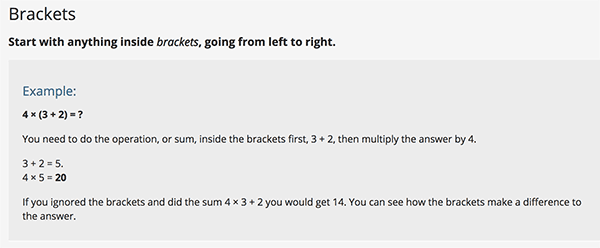## Tuesday, 11 October 2022

### Unit 1 - Ordering operations

Hello, hello!
Today in Maths we're learning the correct order we need to follow when we're solving an equation. We call this: BODMAS.Equations follow a very simple rule:

So, when we want to solve an equation with different operations in it, we need to follow the order:

1. Brackets (the calculation inside the brackets comes first)
2. Orders (numbers involving powers or square roots)
3. Divisions
4. Multiplications
6. Subtractions
Let's have some examples from Skillsyouneed:Let's try to do these together:

Here you also have some games to practice the order of operations:

I hope you find these useful!